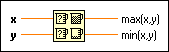# Max & Min Function

FlexRIO Help

Edition Date: November 2015

Part Number: 372614J-01

»View Product Info

Owning Palette: Comparison Functions

Requires: Base Development System

Compares x and y and returns the larger value at the top output terminal and the smaller value at the bottom output terminal. This function accepts time stamp values if all inputs are time stamp values. If the inputs are time stamp values, the function returns the later time at the top and the earlier time at the bottom. You can change the comparison mode of this function.

The connector pane displays the default data types for this polymorphic function.x and y must be of the same type.max(x, y) is the larger value. When you compare arrays in Compare Elements mode (default), this function compares corresponding elements in each input array and returns the element with the maximum value. max(x, y) is an array consisting of the maximum value elements. When you compare arrays in Compare Aggregates mode, this function compares corresponding elements in each input array starting at the beginning of the array. When this function reaches a pair of unequal corresponding elements, max(x, y) returns the array that has the greater value for that element.min(x, y) is the smaller value. When you compare arrays in Compare Elements mode (default), this function compares corresponding elements in each input array and returns the element with the minimum value. min(x, y) is an array consisting of the minimum value elements. When you compare arrays in Compare Aggregates mode, this function compares corresponding elements in each input array starting at the beginning of the array. When this function reaches a pair of unequal corresponding elements, min(x, y) returns the array that has the smaller value for that element.

## Max & Min Details

### Behavior When Comparing NaN Elements

In Compare Elements mode, when one or both inputs are NaN (Not-a-Number), this function produces the following results:

• Both inputs are NaN—Both max(x,y) and min(x,y) return NaN
• Only one input is NaN—Both max(x,y) and min(x,y) return the non-NaN value
• Inputs are arrays—The function evaluates each pair of elements according to the previous rules

In Compare Aggregates mode, when one or both inputs are NaN (Not-a-Number), this function produces the following results:

• Both inputs are NaN—Both max(x,y) and min(x,y) return NaN
• Only one input is NaN—max(x,y) returns x and min(x,y) returns y
• Inputs are arrays—If the function encounters a NaN element in either input array, max(x,y) returns x and min(x,y) returns y

## Example

Refer to the Max and Min VI in the labview\examples\Comparison directory for an example of using the Max & Min function.